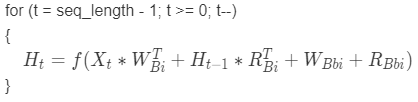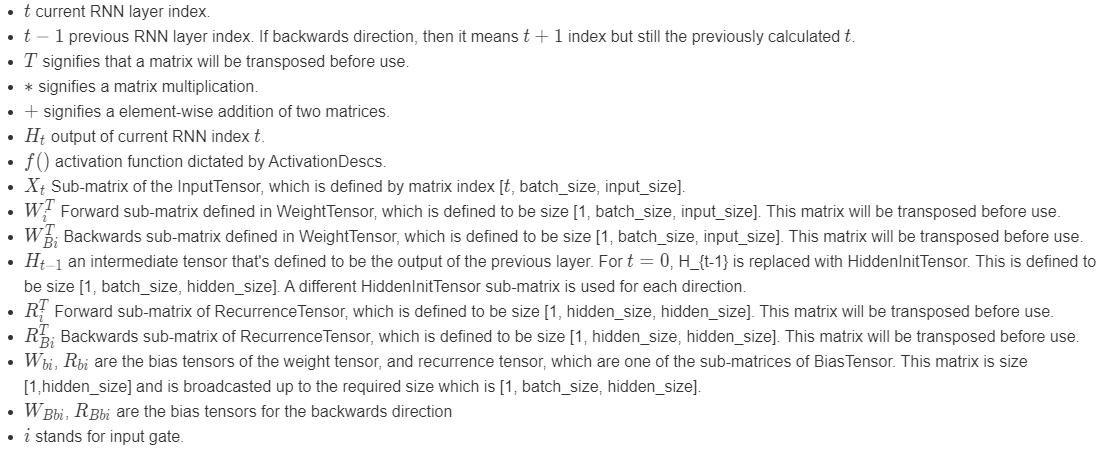# DML_RNN_OPERATOR_DESC structure (directml.h)

Performs a one-layer simple recurrent neural network (RNN) function on the input. This function is often referred to as the Input Gate. This operator performs this function multiple times in a loop, dictated by the sequence length dimension and the SequenceLengthsTensor.

### Equation for the forward direction### Equation for the backward direction### Equation legend## Syntax

``````struct DML_RNN_OPERATOR_DESC {
const DML_TENSOR_DESC           *InputTensor;
const DML_TENSOR_DESC           *WeightTensor;
const DML_TENSOR_DESC           *RecurrenceTensor;
const DML_TENSOR_DESC           *BiasTensor;
const DML_TENSOR_DESC           *HiddenInitTensor;
const DML_TENSOR_DESC           *SequenceLengthsTensor;
const DML_TENSOR_DESC           *OutputSequenceTensor;
const DML_TENSOR_DESC           *OutputSingleTensor;
UINT                            ActivationDescCount;
const DML_OPERATOR_DESC         *ActivationDescs;
DML_RECURRENT_NETWORK_DIRECTION Direction;
};
``````

## Members

`InputTensor`

Type: const DML_TENSOR_DESC*

A tensor containing the input data, X. Packed (and potentially padded) into one 4-D tensor with the sizes of `{ 1, seq_length, batch_size, input_size }`. seq_length is the dimension that is mapped to the index, t.

`WeightTensor`

Type: const DML_TENSOR_DESC*

A tensor containing the weight data, W. Concatenation of W_i and W_Bi (if bidirectional). The tensor has sizes `{ 1, num_directions, hidden_size, input_size }`.

`RecurrenceTensor`

Type: const DML_TENSOR_DESC*

An optional tensor containing the recurrence weight data, R. Concatenation of R_i and R_Bi (if bidirectional). This tensor has sizes `{ 1, num_directions, hidden_size, hidden_size }`.

`BiasTensor`

Type: _Maybenull_ const DML_TENSOR_DESC*

An optional tensor containing the bias data for the input gate, B. Concatenation of `{ W_bi, R_bi }`, and `{ W_Bbi, R_Bbi }` (if bidirectional). This tensor has sizes `{ 1, 1, num_directions, 2 * hidden_size }`. If not specified, then defaults to 0.

`HiddenInitTensor`

Type: _Maybenull_ const DML_TENSOR_DESC*

An optional tensor containing the hidden node initializer tensor, H_[t-1] for the first loop index t. If not specified, then defaults to 0. This tensor has sizes `{ 1, num_directions, batch_size, hidden_size }`.

`SequenceLengthsTensor`

Type: _Maybenull_ const DML_TENSOR_DESC*

An optional tensor containing an independent seq_length for each element in the batch. If not specified, then all sequences in the batch have length seq_length. This tensor has sizes `{ 1, 1, 1, batch_size }`.

`OutputSequenceTensor`

Type: _Maybenull_ const DML_TENSOR_DESC*

An optional tensor with which to write the concatenation of all the intermediate layer output values of the hidden nodes, H_t. This tensor has sizes `{ seq_length, num_directions, batch_size, hidden_size }`. seq_length is mapped to the loop index t.

`OutputSingleTensor`

Type: _Maybenull_ const DML_TENSOR_DESC*

An optional tensor with which to write the final output value of the hidden nodes, H_t. This tensor has sizes `{ 1, num_directions, batch_size, hidden_size }`.

`ActivationDescCount`

Type: UINT

This field determines the size of the ActivationDescs array.

`ActivationDescs`

Type: _Field_size_(ActivationDescCount) const DML_OPERATOR_DESC*

An array of DML_OPERATOR_DESC containing the descriptions of the activation operators, f(). The number of activation functions is equal to the number of directions. For forwards and backwards directions there is expected to be 1 activation function. For Bidirectional there are expected to be 2.

`Direction`

The direction of the operator: forward, backward, or bidirectional.

## Availability

This operator was introduced in `DML_FEATURE_LEVEL_1_0`.

## Tensor constraints

BiasTensor, `HiddenInitTensor`, InputTensor, `OutputSequenceTensor`, `OutputSingleTensor`, `RecurrenceTensor`, and `WeightTensor` must have the same DataType.

## Tensor support

Tensor Kind Supported dimension counts Supported data types
InputTensor Input 4 FLOAT32, FLOAT16
WeightTensor Input 4 FLOAT32, FLOAT16
RecurrenceTensor Input 4 FLOAT32, FLOAT16
BiasTensor Optional input 4 FLOAT32, FLOAT16
HiddenInitTensor Optional input 4 FLOAT32, FLOAT16
SequenceLengthsTensor Optional input 4 UINT32
OutputSequenceTensor Optional output 4 FLOAT32, FLOAT16
OutputSingleTensor Optional output 4 FLOAT32, FLOAT16